# RS Aggarwal Solutions for Class 6 Maths Chapter 21 Concept of Perimeter and Area

RS Aggarwal Solutions for Class 6 Chapter 21 Concepts of Perimeter and Area are given in simple PDF. Students who want to understand this chapter effectively are advised to practice RS Aggarwal textbook questions regularly. Practicing RS Aggarwal textbook will boost confidence in students and help them to perform well in examination. Students who want to understand the Concept of Perimeter and Area in depth should refer RS Aggarwal Solutions for Class 6 Chapter 21 and solve them.

PDF format of RS Aggarwal textbook questions have been solved by BYJU’S experts in Mathematics. Download PDF of Class 6 Chapter 21 in their respective links.

## Download PDF of RS Aggarwal Solutions for Class 6 Chapter 21 Concept of Perimeter and Area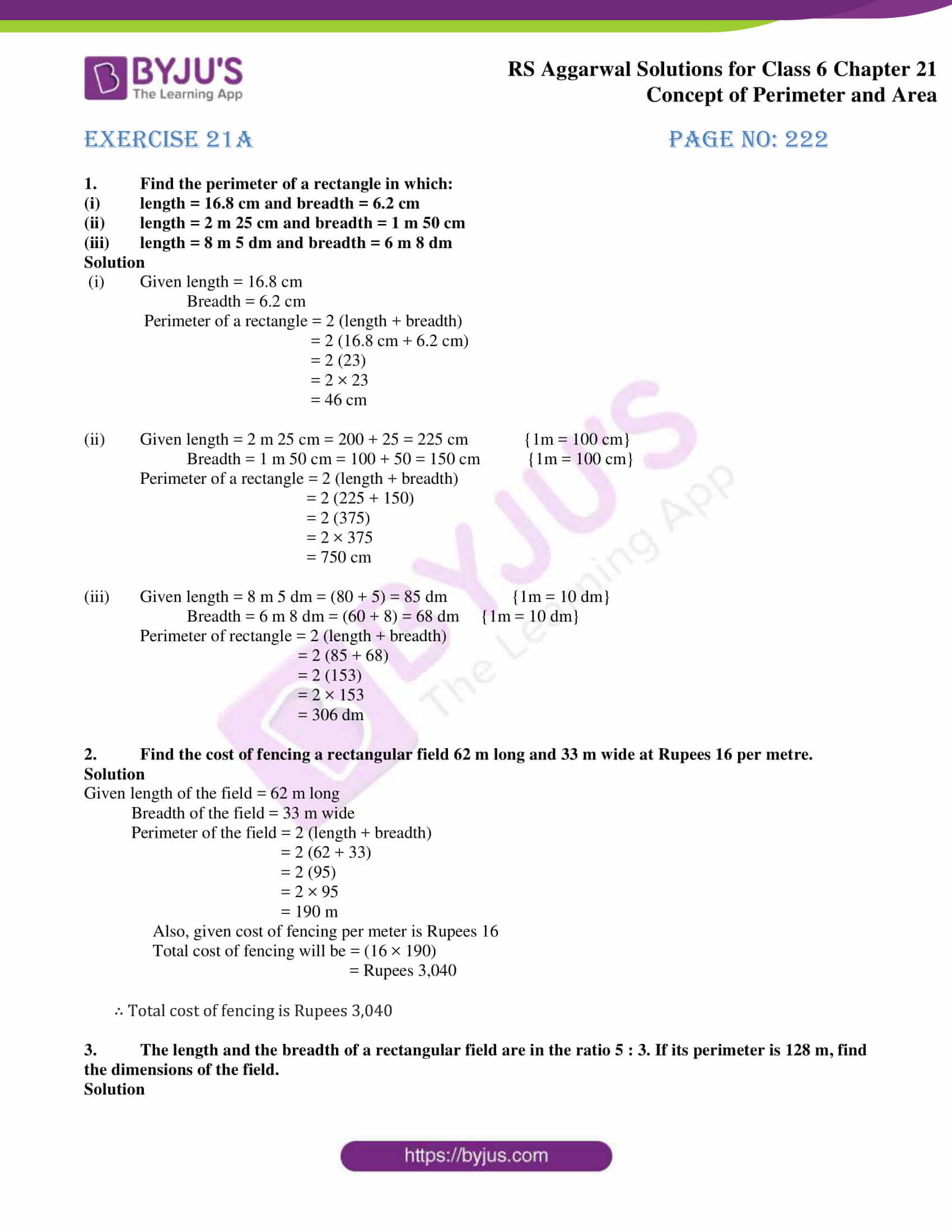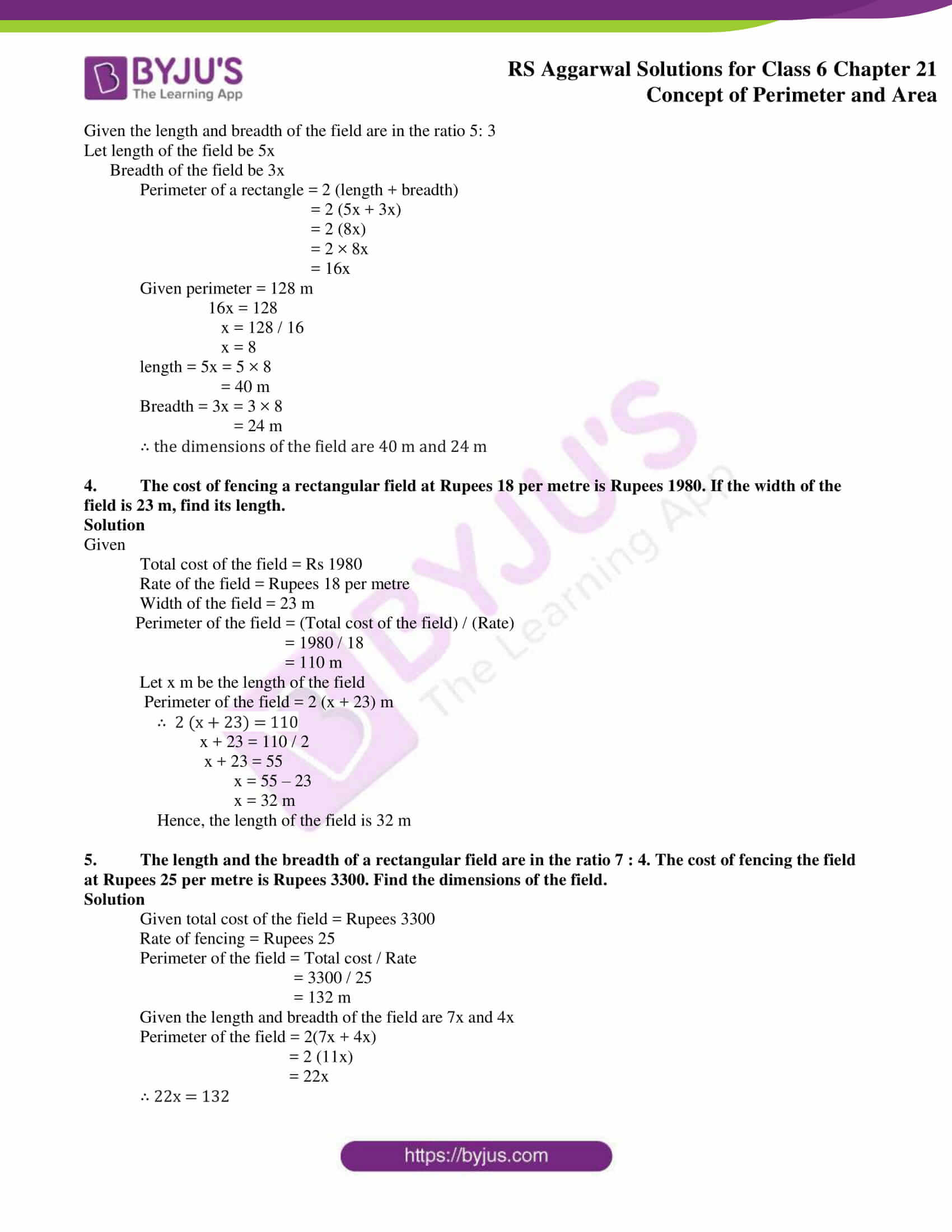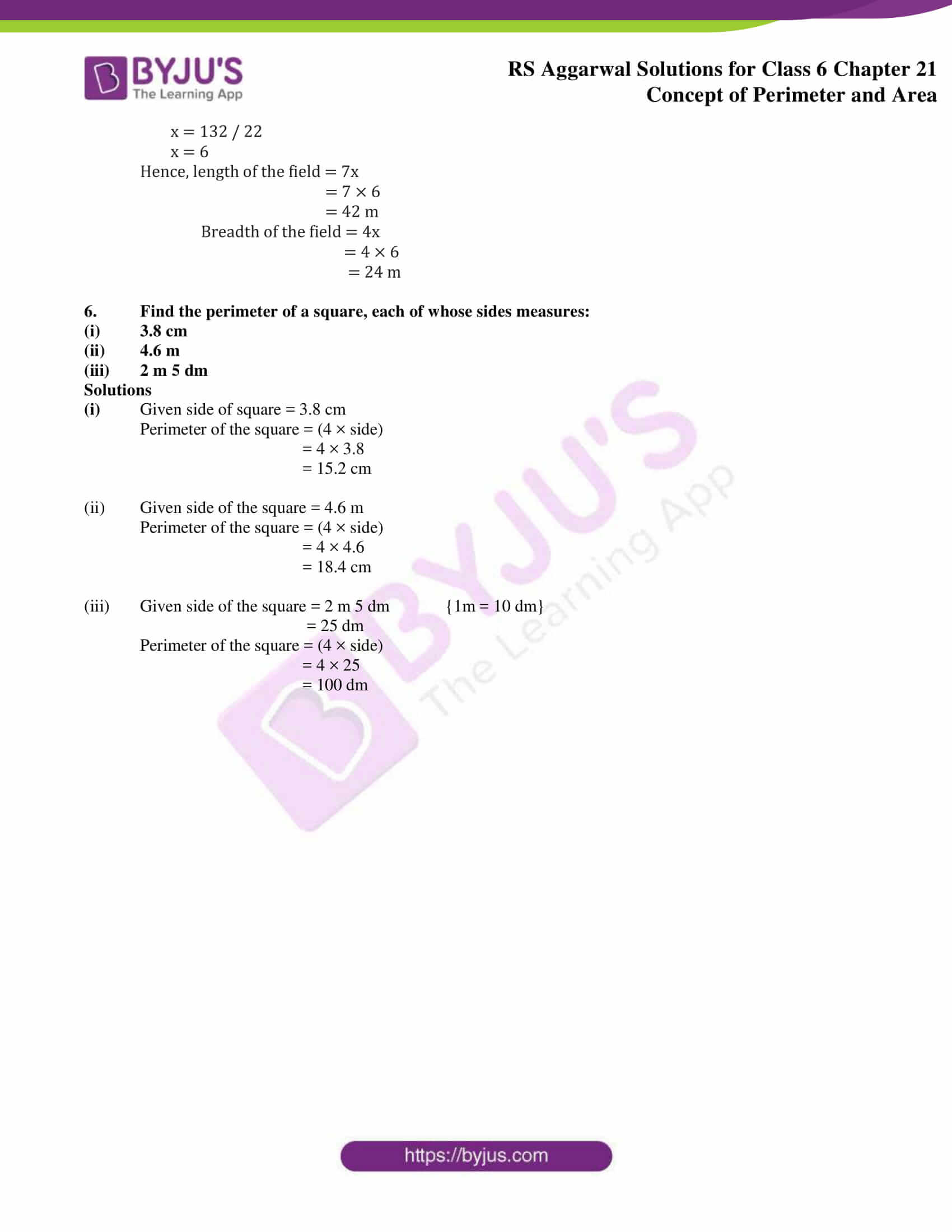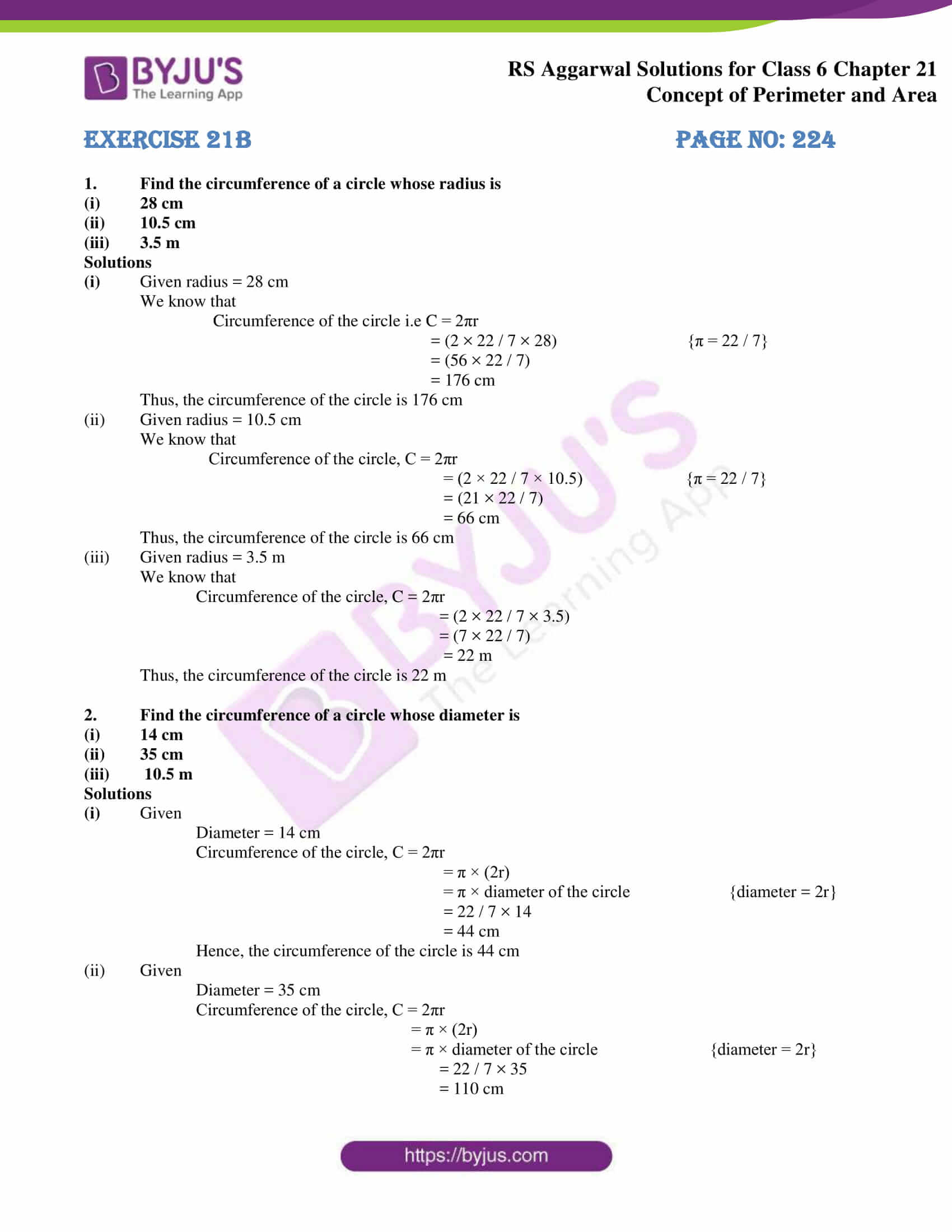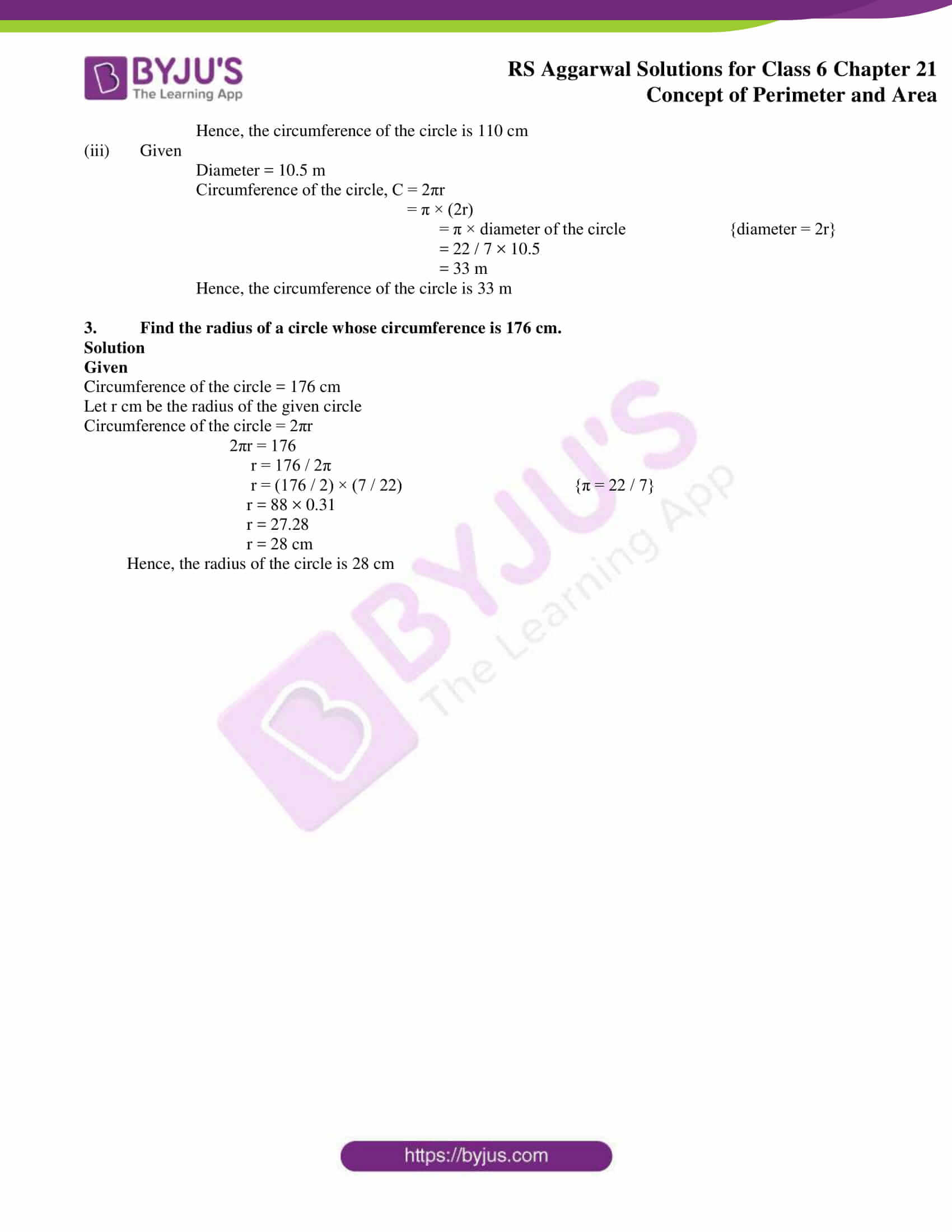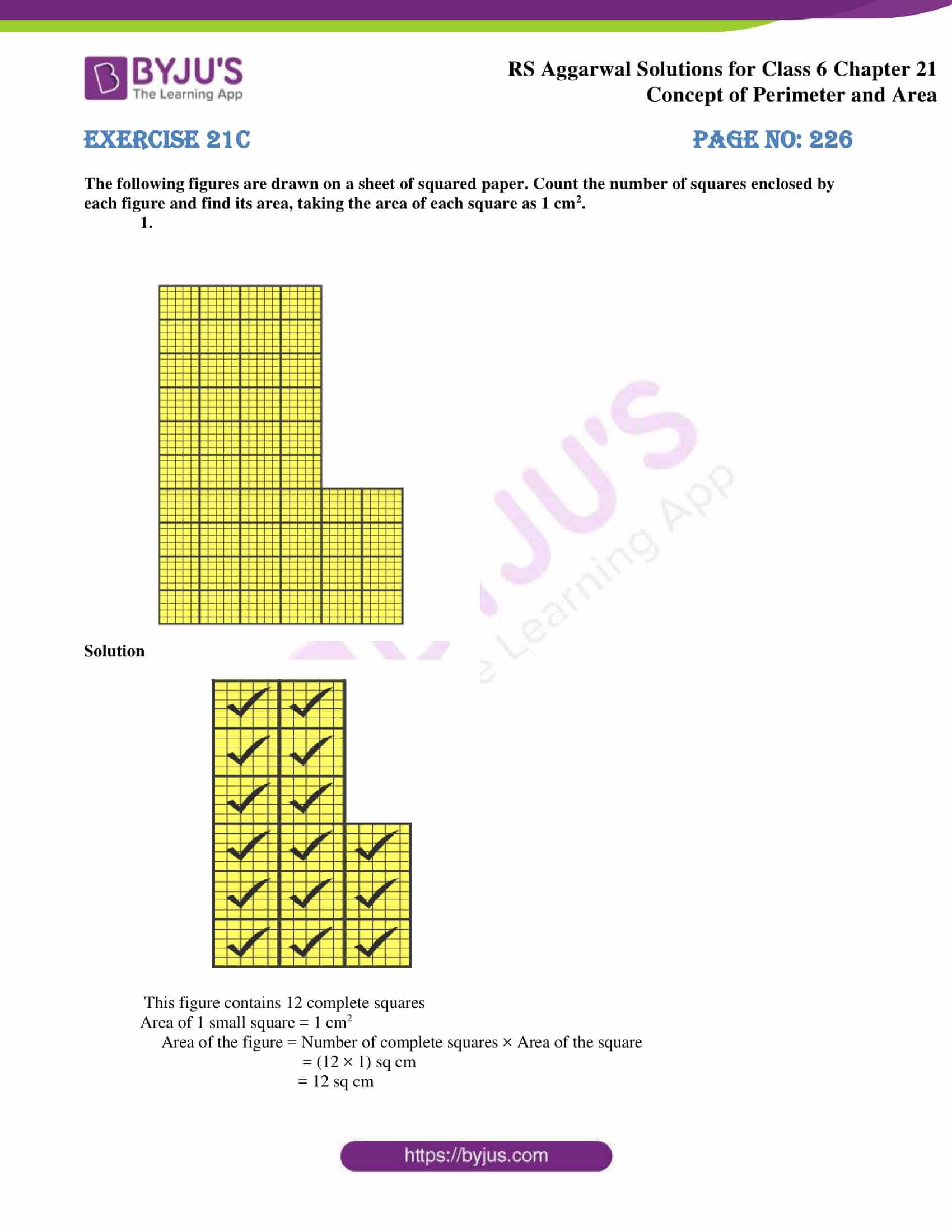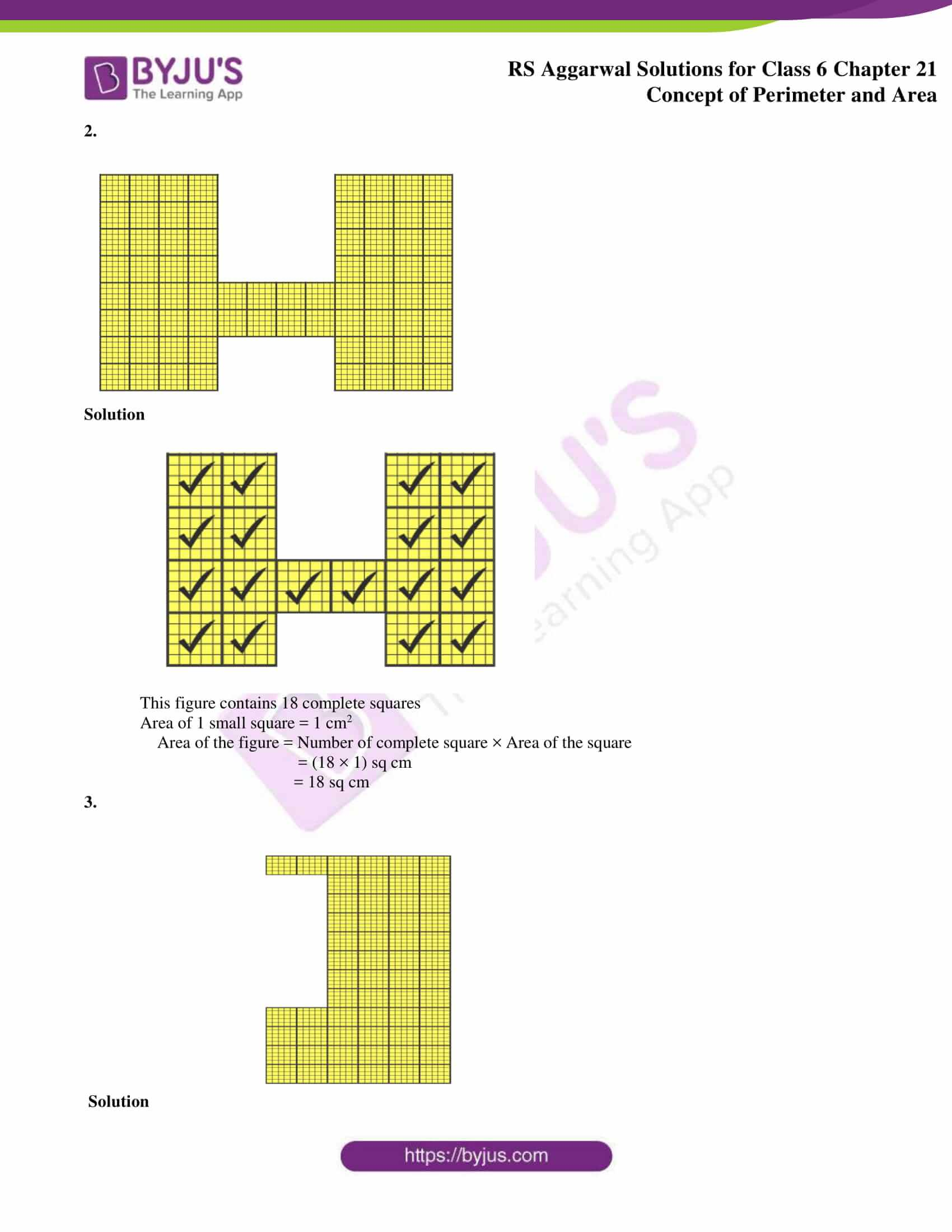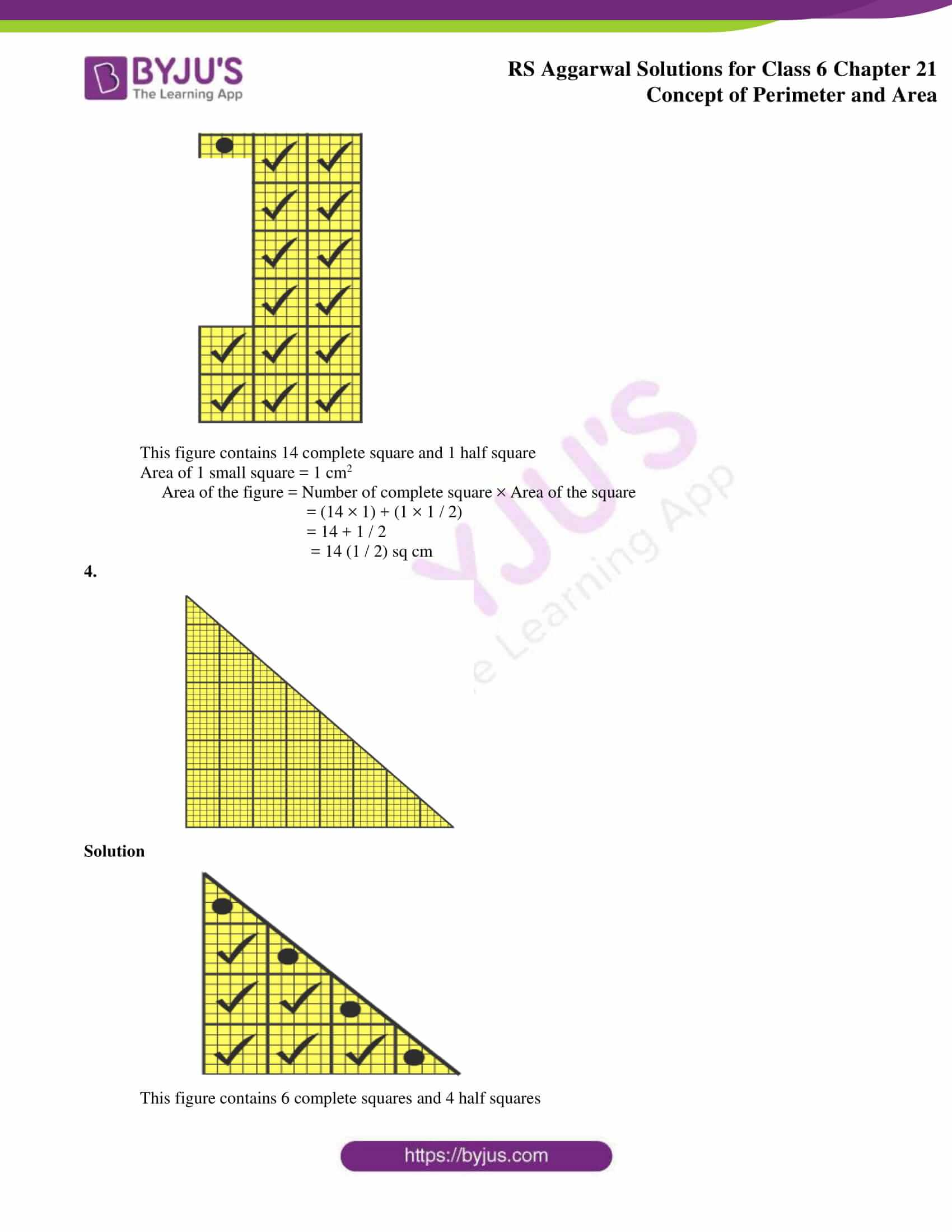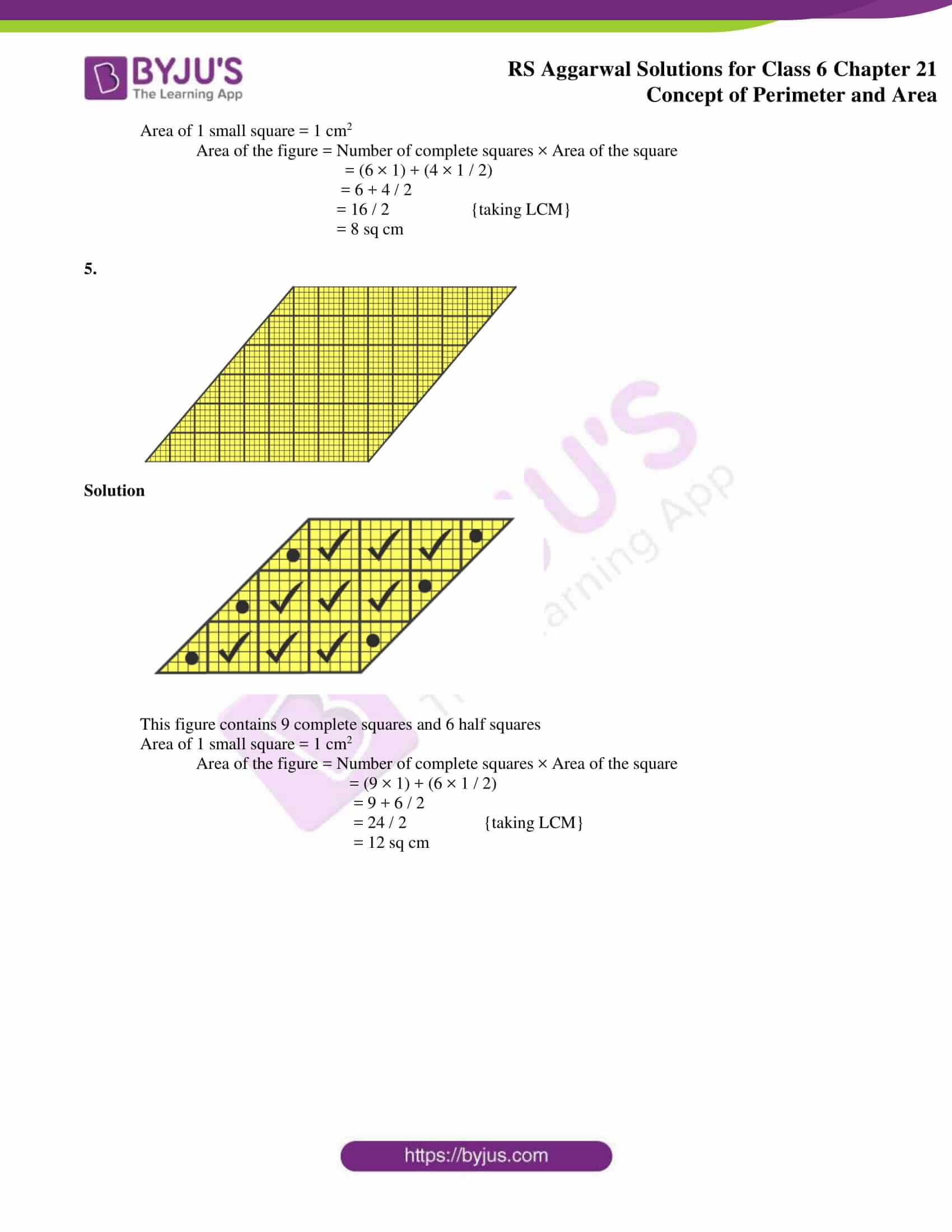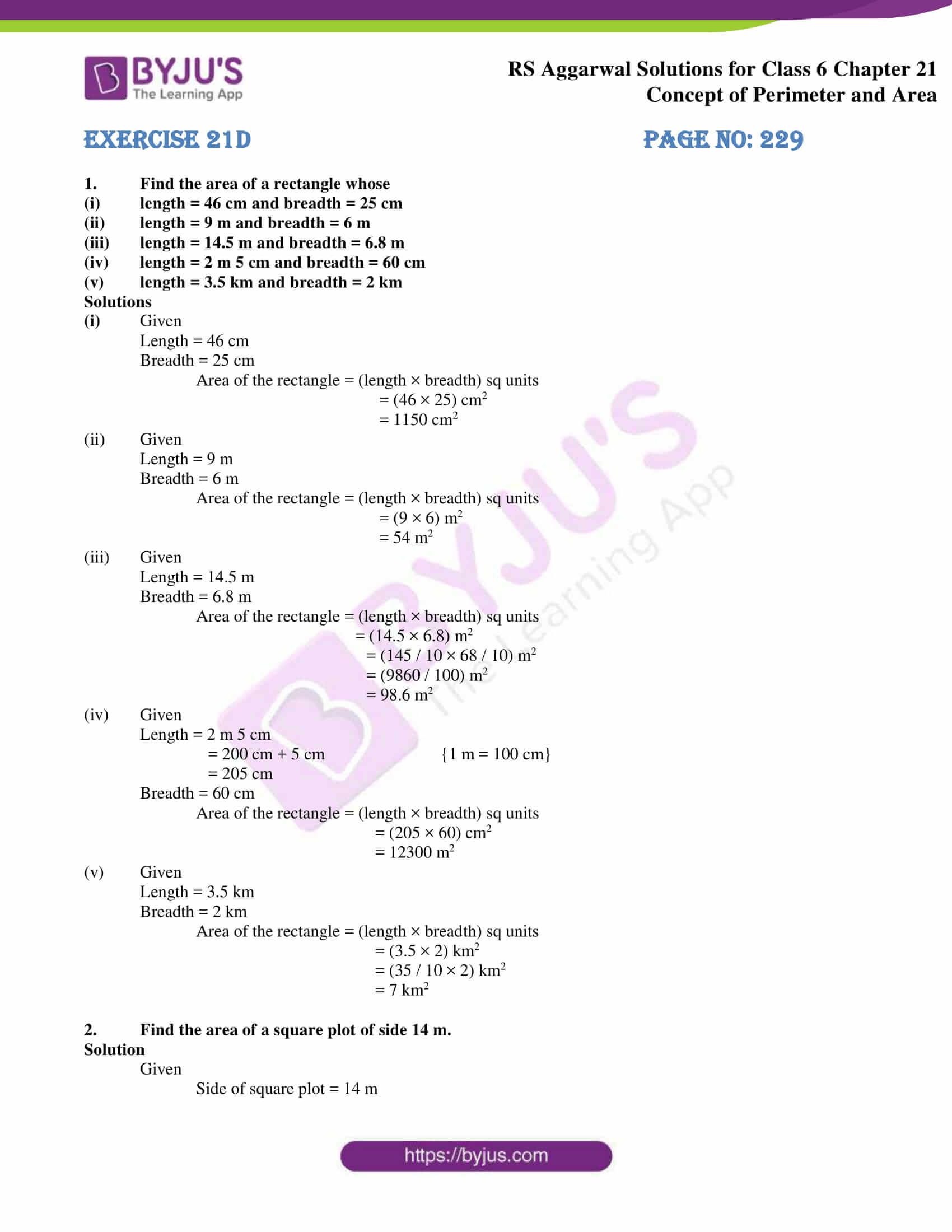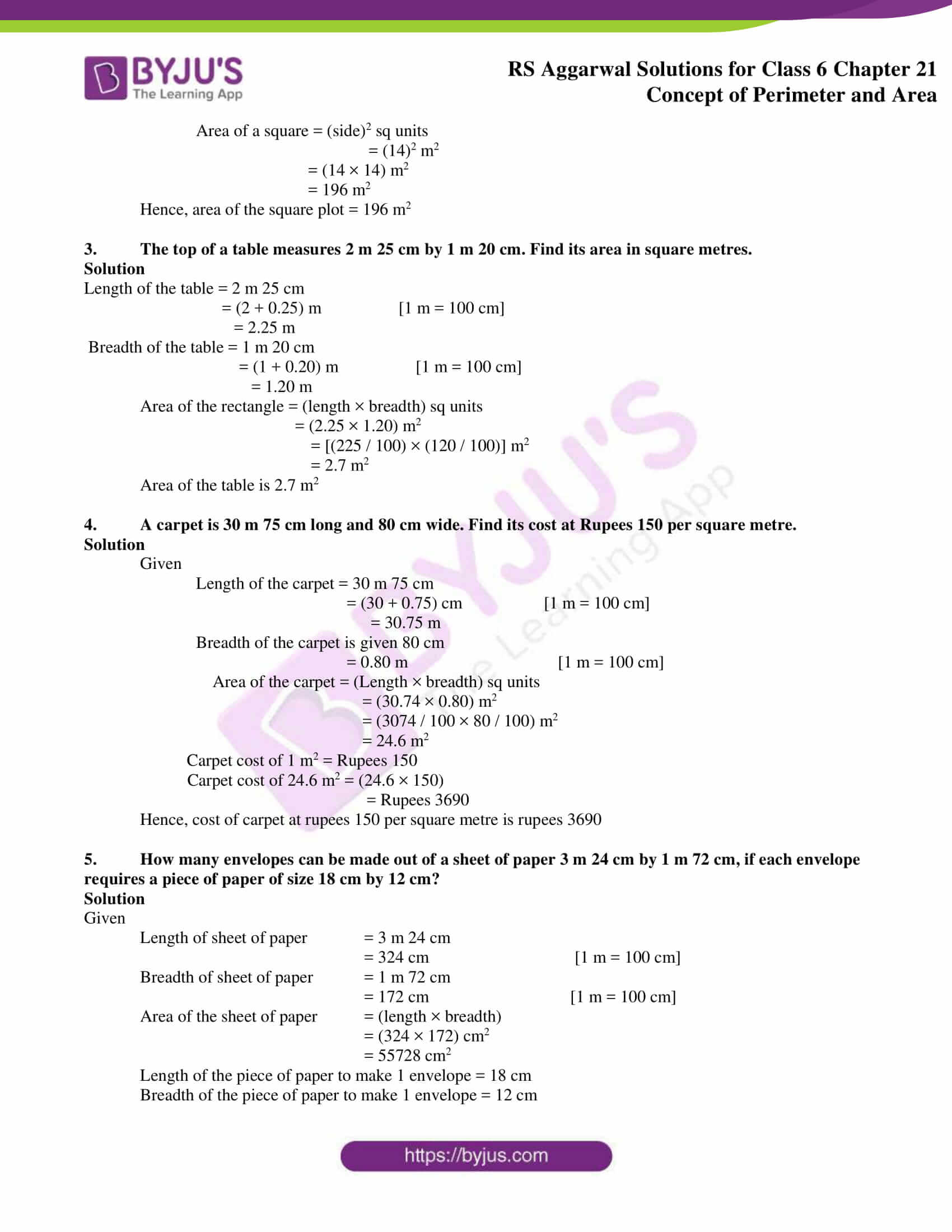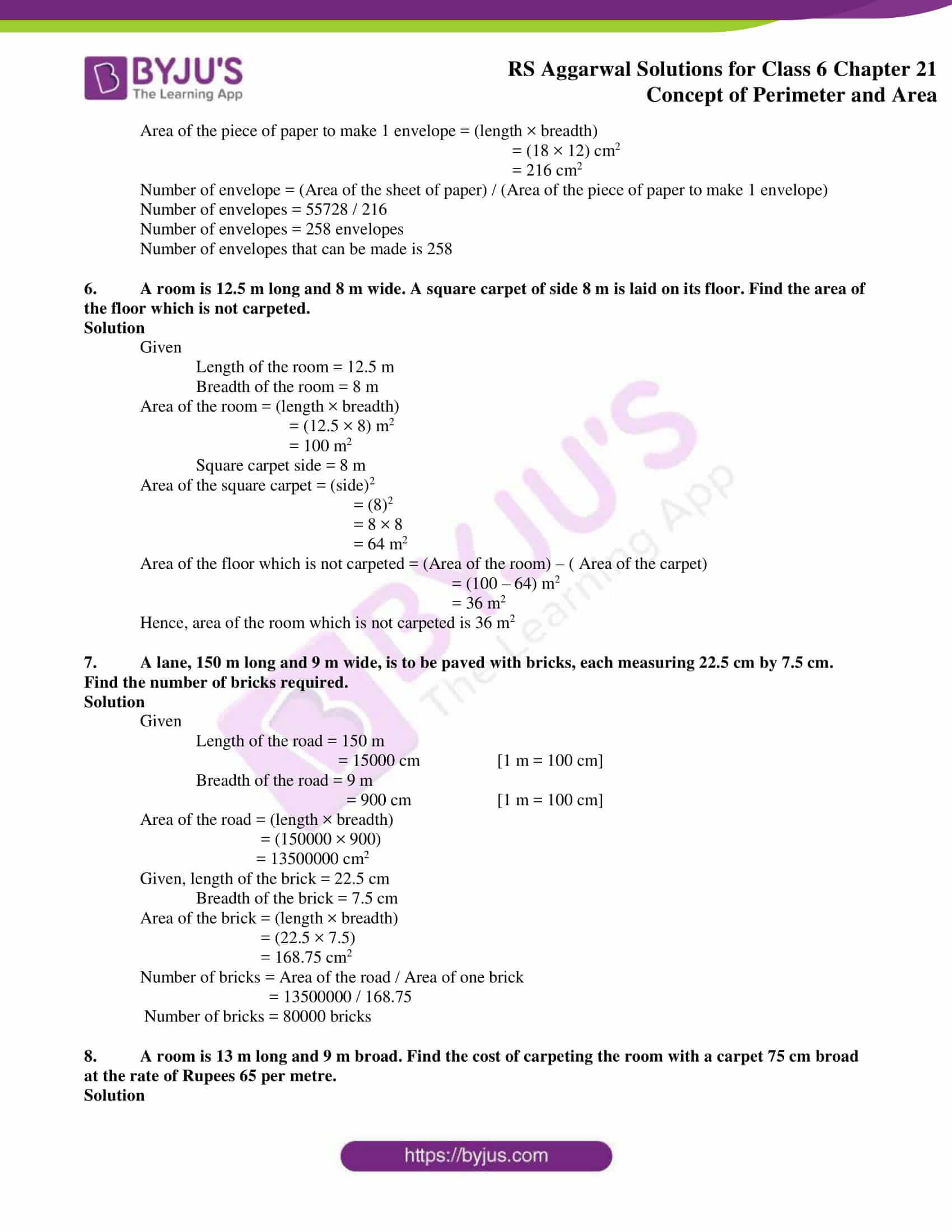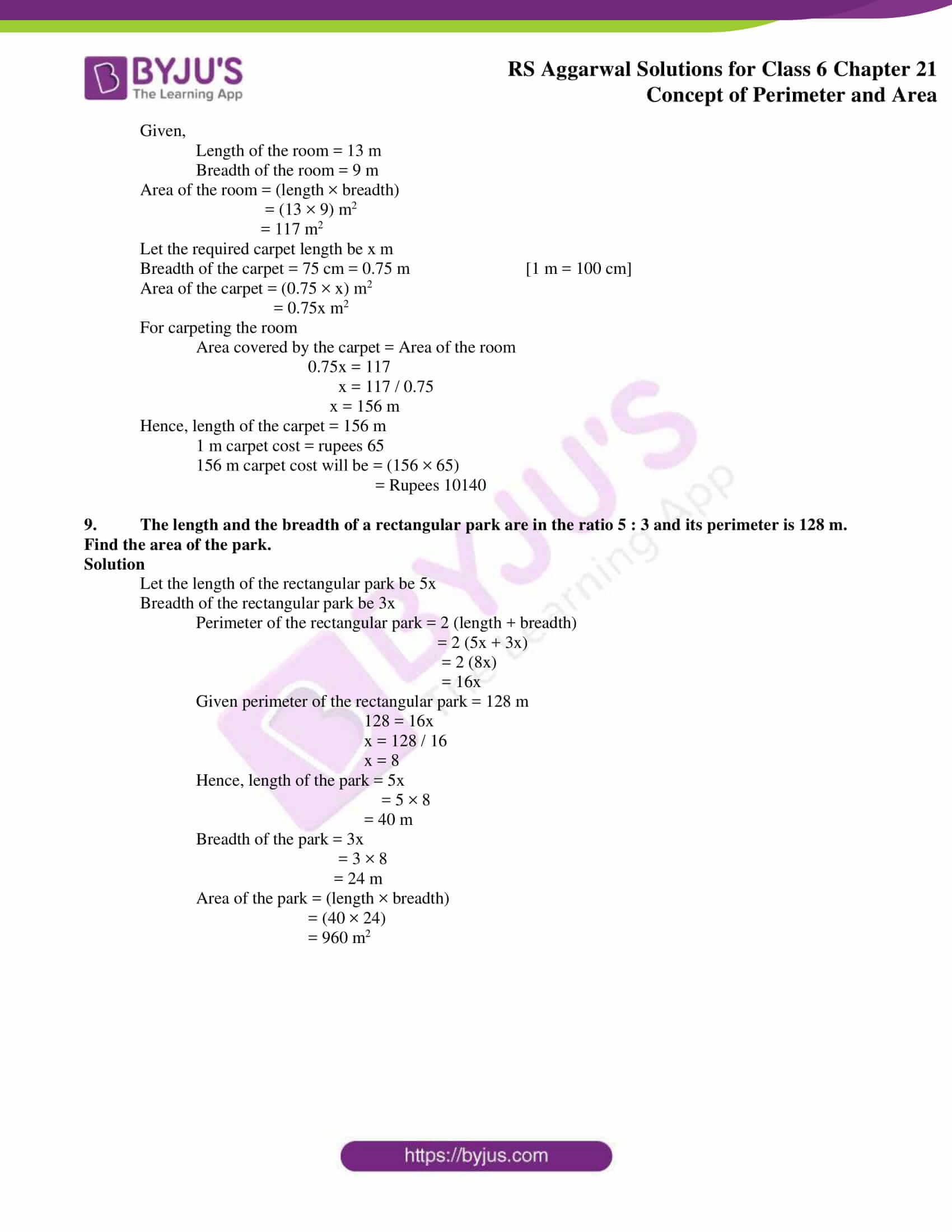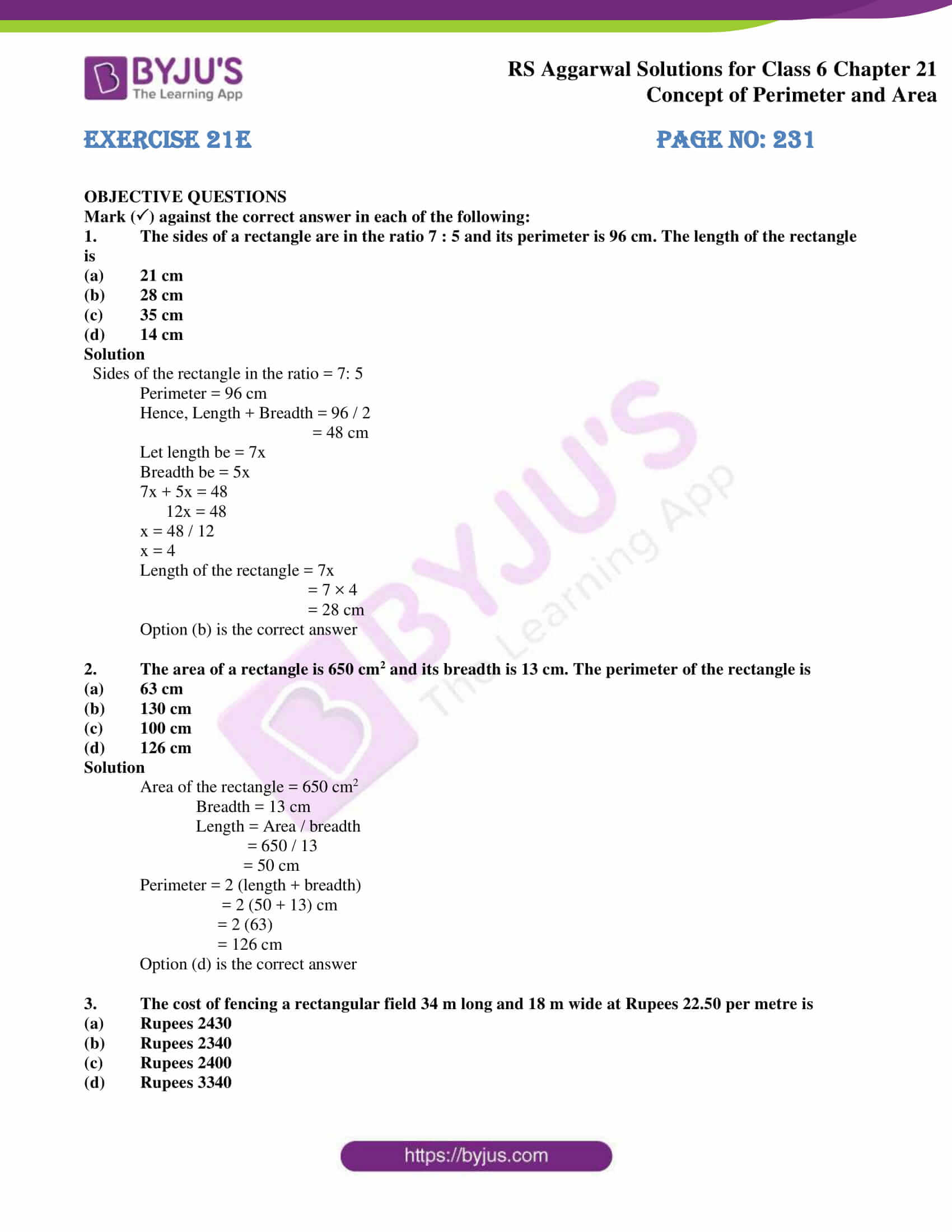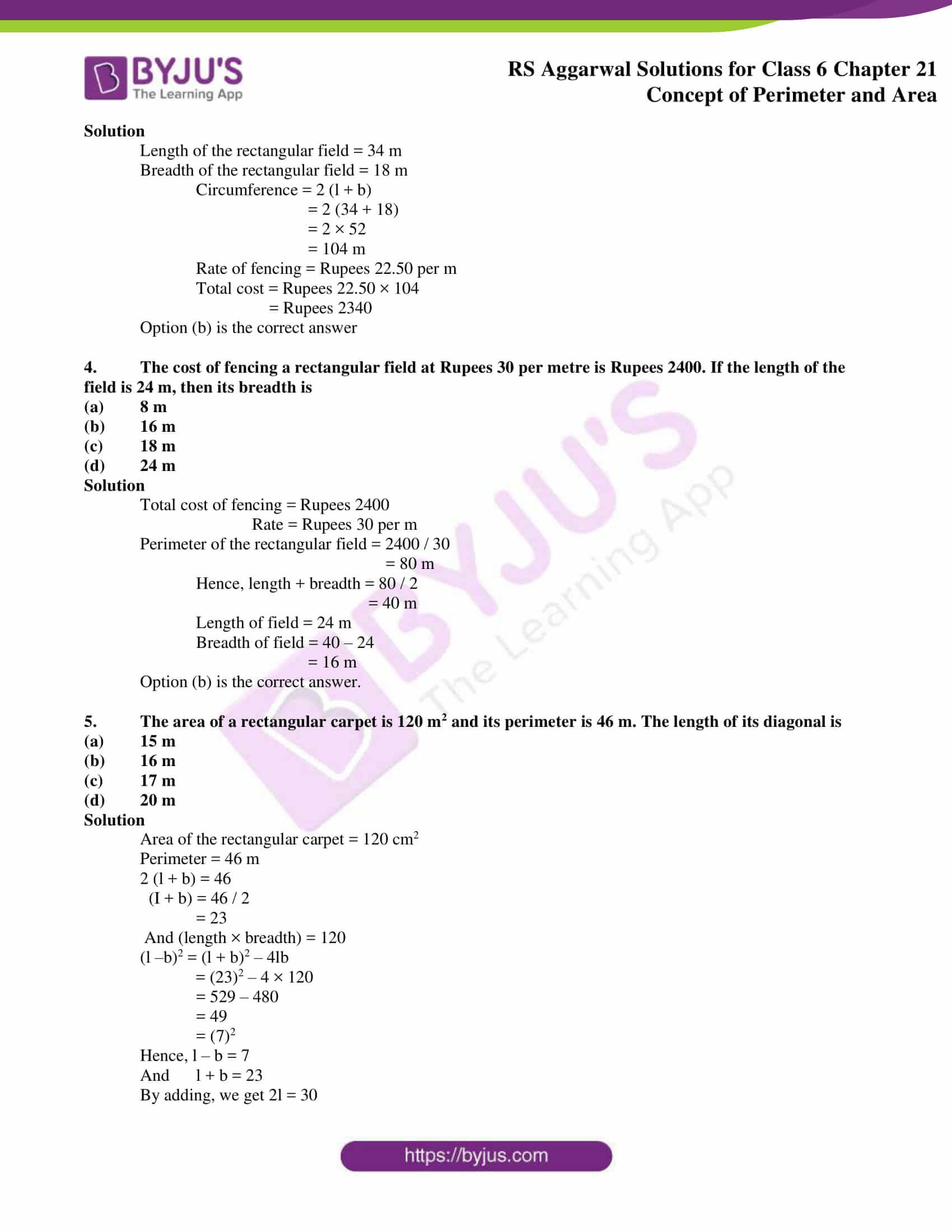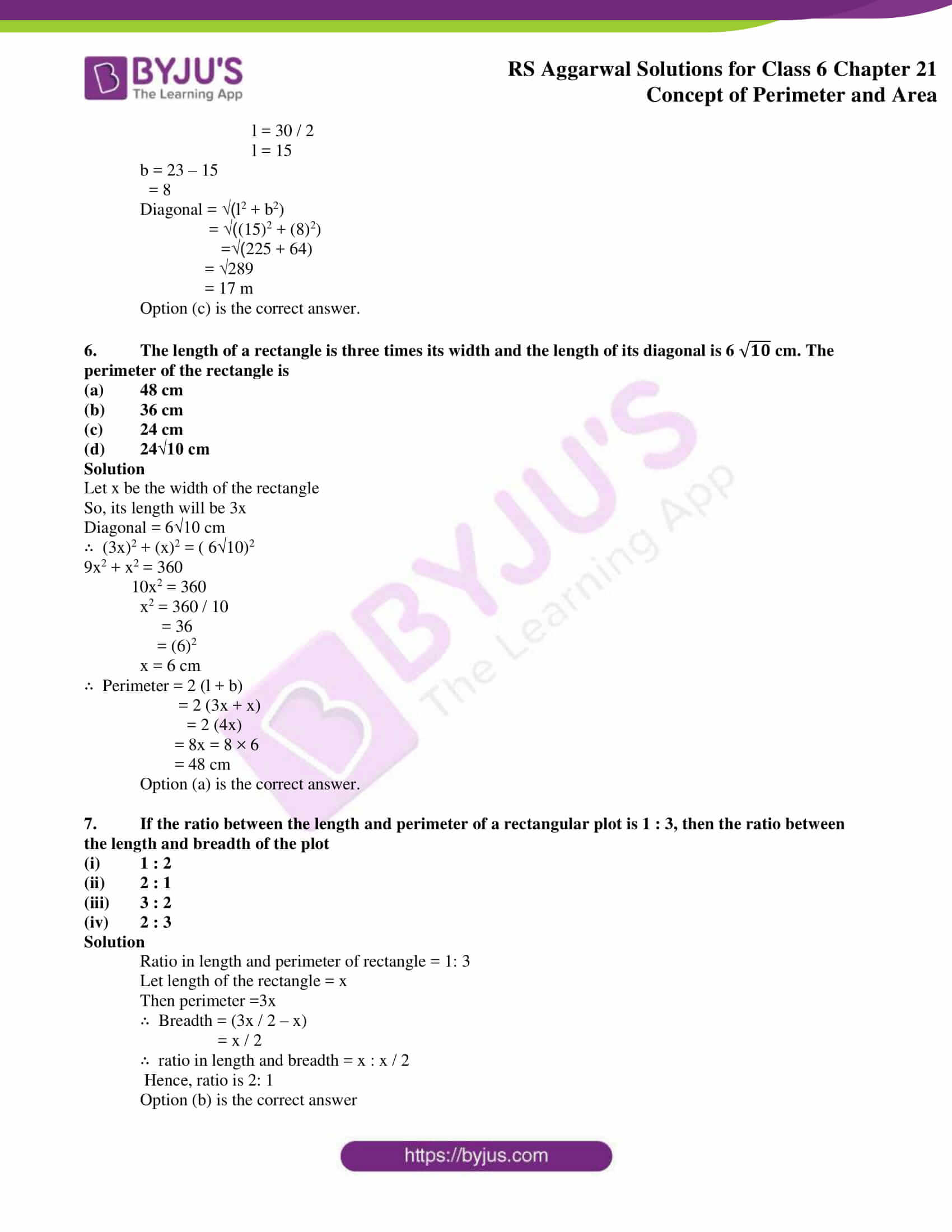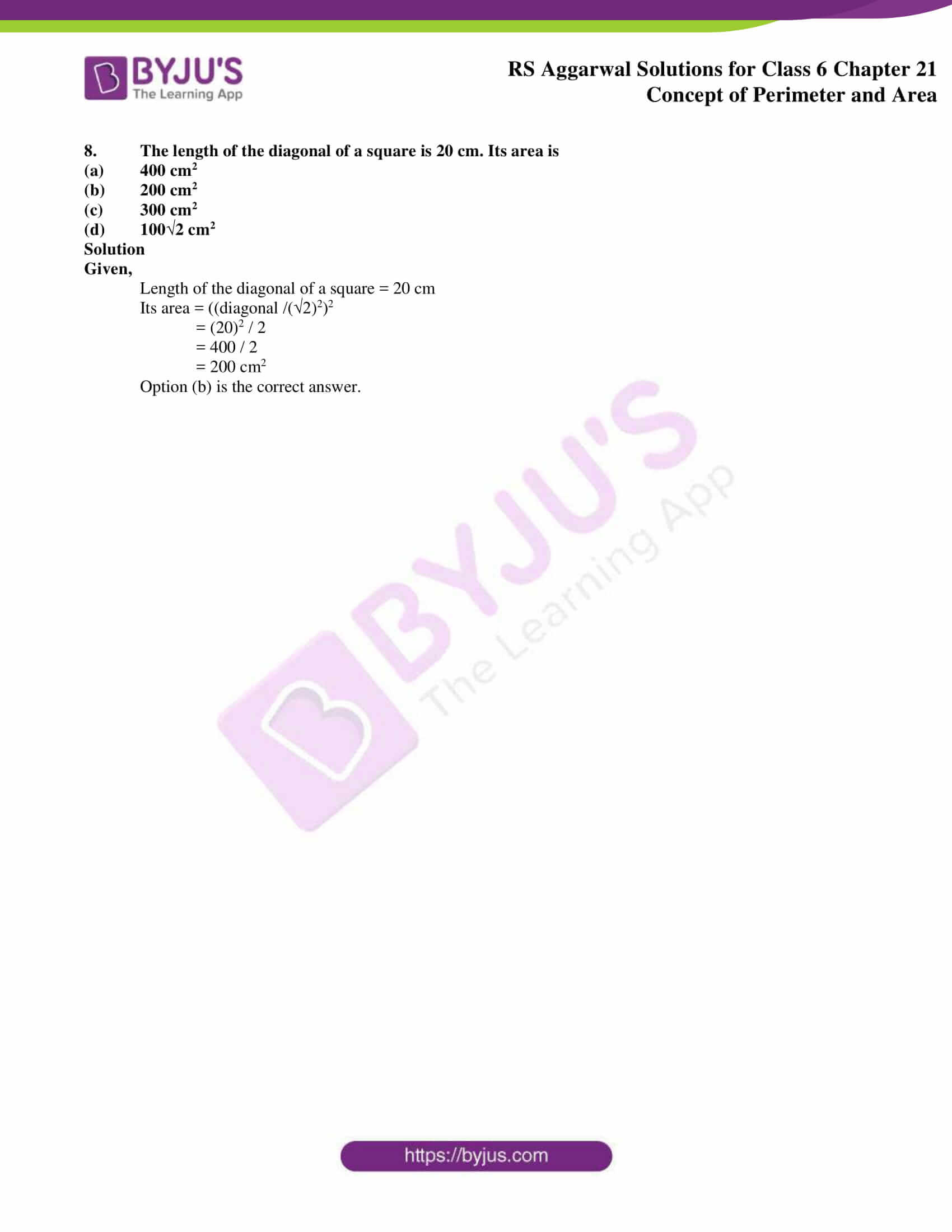## Exercise 21A PAGE NO: 222

1. Find the perimeter of a rectangle in which:

(i) length = 16.8 cm and breadth = 6.2 cm

(ii) length = 2 m 25 cm and breadth = 1 m 50 cm

(iii) length = 8 m 5 dm and breadth = 6 m 8 dm

Solution

(i) Given length = 16.8 cm

Perimeter of a rectangle = 2 (length + breadth)

= 2 (16.8 cm + 6.2 cm)

= 2 (23)

= 2 × 23

= 46 cm

(ii) Given length = 2 m 25 cm = 200 + 25 = 225 cm {1m = 100 cm}

Breadth = 1 m 50 cm = 100 + 50 = 150 cm {1m = 100 cm}

Perimeter of a rectangle = 2 (length + breadth)

= 2 (225 + 150)

= 2 (375)

= 2 × 375

= 750 cm

(iii) Given length = 8 m 5 dm = (80 + 5) = 85 dm {1m = 10 dm}

Breadth = 6 m 8 dm = (60 + 8) = 68 dm {1m = 10 dm}

Perimeter of rectangle = 2 (length + breadth)

= 2 (85 + 68)

= 2 (153)

= 2 × 153

= 306 dm

2. Find the cost of fencing a rectangular field 62 m long and 33 m wide at Rupees 16 per metre.

Solution

Given length of the field = 62 m long

Breadth of the field = 33 m wide

Perimeter of the field = 2 (length + breadth)

= 2 (62 + 33)

= 2 (95)

= 2 × 95

= 190 m

Also, given cost of fencing per meter is Rupees 16

Total cost of fencing will be = (16 × 190)

= Rupees 3,040

∴ Total cost of fencing is Rupees 3,040

3. The length and the breadth of a rectangular field are in the ratio 5 : 3. If its perimeter is 128 m, find the dimensions of the field.

Solution

Given the length and breadth of the field are in the ratio 5: 3

Let length of the field be 5x

Breadth of the field be 3x

Perimeter of a rectangle = 2 (length + breadth)

= 2 (5x + 3x)

= 2 (8x)

= 2 × 8x

= 16x

Given perimeter = 128 m

16x = 128

x = 128 / 16

x = 8

length = 5x = 5 × 8

= 40 m

Breadth = 3x = 3 × 8

= 24 m

∴ the dimensions of the field are 40 m and 24 m

4. The cost of fencing a rectangular field at Rupees 18 per metre is Rupees 1980. If the width of the field is 23 m, find its length.

Solution

Given

Total cost of the field = Rs 1980

Rate of the field = Rupees 18 per metre

Width of the field = 23 m

Perimeter of the field = (Total cost of the field) / (Rate)

= 1980 / 18

= 110 m

Let x m be the length of the field

Perimeter of the field = 2 (x + 23) m

∴ 2 (x + 23) = 110

x + 23 = 110 / 2

x + 23 = 55

x = 55 – 23

x = 32 m

Hence, the length of the field is 32 m

5. The length and the breadth of a rectangular field are in the ratio 7 : 4. The cost of fencing the field at Rupees 25 per metre is Rupees 3300. Find the dimensions of the field.

Solution

Given total cost of the field = Rupees 3300

Rate of fencing = Rupees 25

Perimeter of the field = Total cost / Rate

= 3300 / 25

= 132 m

Given the length and breadth of the field are 7x and 4x

Perimeter of the field = 2(7x + 4x)

= 2 (11x)

= 22x

∴ 22x = 132

x = 132 / 22

x = 6

Hence, length of the field = 7x

= 7 × 6

= 42 m

Breadth of the field = 4x

= 4 × 6

= 24 m

6. Find the perimeter of a square, each of whose sides measures:

(i) 3.8 cm

(ii) 4.6 m

(iii) 2 m 5 dm

Solutions

(i) Given side of square = 3.8 cm

Perimeter of the square = (4 × side)

= 4 × 3.8

= 15.2 cm

(ii) Given side of the square = 4.6 m

Perimeter of the square = (4 × side)

= 4 × 4.6

= 18.4 cm

(iii) Given side of the square = 2 m 5 dm {1m = 10 dm}

= 25 dm

Perimeter of the square = (4 × side)

= 4 × 25

= 100 dm

Exercise 21B PAGE NO: 224

1. Find the circumference of a circle whose radius is

(i) 28 cm

(ii) 10.5 cm

(iii) 3.5 m

Solutions

(i) Given radius = 28 cm

We know that

Circumference of the circle i.e C = 2πr

= (2 × 22 / 7 × 28) {π = 22 / 7}

= (56 × 22 / 7)

= 176 cm

Thus, the circumference of the circle is 176 cm

(ii) Given radius = 10.5 cm

We know that

Circumference of the circle, C = 2πr

= (2 × 22 / 7 × 10.5) {π = 22 / 7}

= (21 × 22 / 7)

= 66 cm

Thus, the circumference of the circle is 66 cm

(iii) Given radius = 3.5 m

We know that

Circumference of the circle, C = 2πr

= (2 × 22 / 7 × 3.5)

= (7 × 22 / 7)

= 22 m

Thus, the circumference of the circle is 22 m

2. Find the circumference of a circle whose diameter is

(i) 14 cm

(ii) 35 cm

(iii) 10.5 m

Solutions

(i) Given

Diameter = 14 cm

Circumference of the circle, C = 2πr

= π × (2r)

= π × diameter of the circle {diameter = 2r}

= 22 / 7 × 14

= 44 cm

Hence, the circumference of the circle is 44 cm

(ii) Given

Diameter = 35 cm

Circumference of the circle, C = 2πr

= π × (2r)

= π × diameter of the circle {diameter = 2r}

= 22 / 7 × 35

= 110 cm

Hence, the circumference of the circle is 110 cm

(iii) Given

Diameter = 10.5 m

Circumference of the circle, C = 2πr

= π × (2r)

= π × diameter of the circle {diameter = 2r}

= 22 / 7 × 10.5

= 33 m

Hence, the circumference of the circle is 33 m

3. Find the radius of a circle whose circumference is 176 cm.

Solution

Given

Circumference of the circle = 176 cm

Let r cm be the radius of the given circle

Circumference of the circle = 2πr

2πr = 176

r = 176 / 2π

r = (176 / 2) × (7 / 22) {π = 22 / 7}

r = 88 × 0.31

r = 27.28

r = 28 cm

Hence, the radius of the circle is 28 cm

Exercise 21C PAGE NO: 226

The following figures are drawn on a sheet of squared paper. Count the number of squares enclosed by each figure and find its area, taking the area of each square as 1 cm2.

1.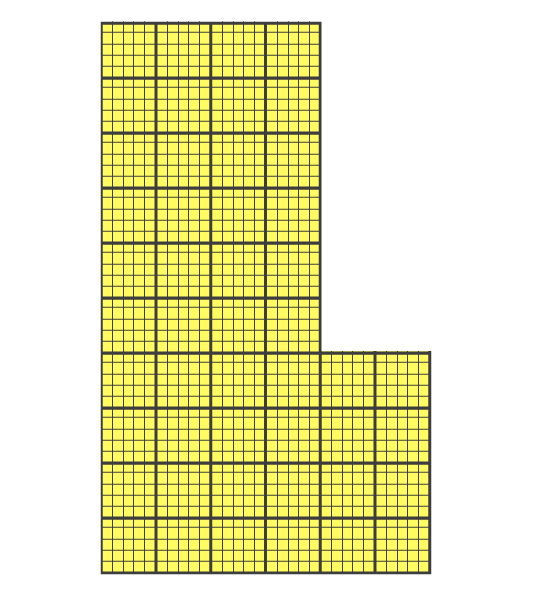Solution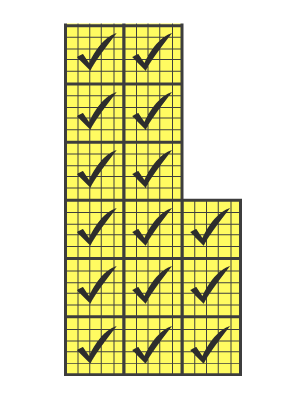This figure contains 12 complete squares

Area of 1 small square = 1 cm2

Area of the figure = Number of complete squares × Area of the square

= (12 × 1) sq cm

= 12 sq cm

2.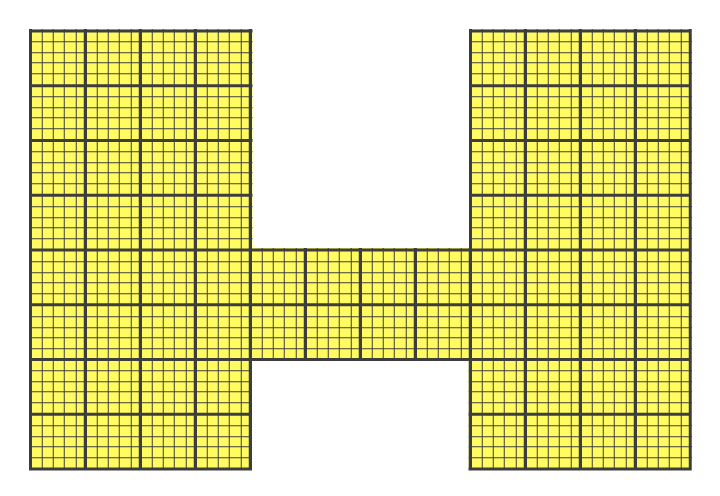Solution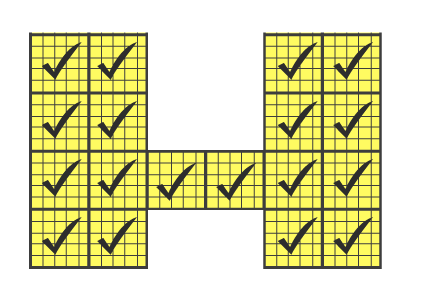This figure contains 18 complete squares

Area of 1 small square = 1 cm2

Area of the figure = Number of complete square × Area of the square

= (18 × 1) sq cm

= 18 sq cm

3.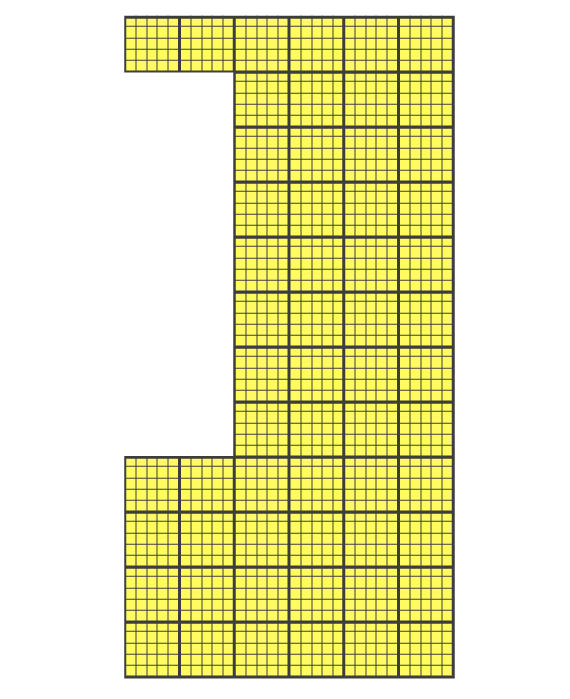Solution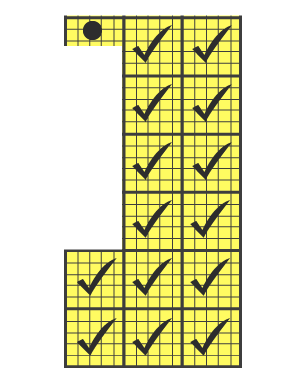This figure contains 14 complete square and 1 half square

Area of 1 small square = 1 cm2

Area of the figure = Number of complete square × Area of the square

= (14 × 1) + (1 × 1 / 2)

= 14 + 1 / 2

= 14 (1 / 2) sq cm

4.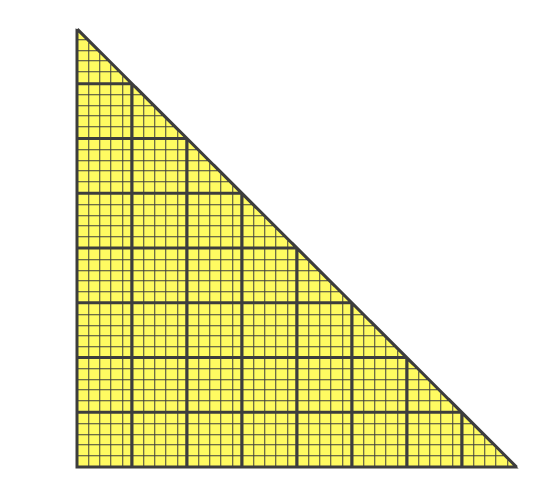Solution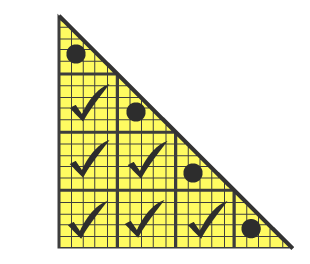This figure contains 6 complete squares and 4 half squares

Area of 1 small square = 1 cm2

Area of the figure = Number of complete squares × Area of the square

= (6 × 1) + (4 × 1 / 2)

= 6 + 4 / 2

= 16 / 2 {taking LCM}

= 8 sq cm

5.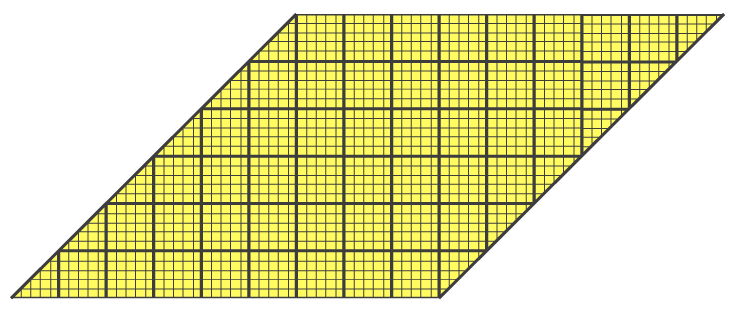Solution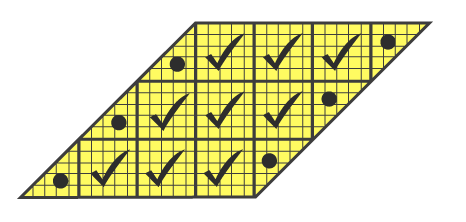This figure contains 9 complete squares and 6 half squares

Area of 1 small square = 1 cm2

Area of the figure = Number of complete squares × Area of the square

= (9 × 1) + (6 × 1 / 2)

= 9 + 6 / 2

= 24 / 2 {taking LCM}

= 12 sq cm

Exercise 21D PAGE NO: 229

1. Find the area of a rectangle whose

(i) length = 46 cm and breadth = 25 cm

(ii) length = 9 m and breadth = 6 m

(iii) length = 14.5 m and breadth = 6.8 m

(iv) length = 2 m 5 cm and breadth = 60 cm

(v) length = 3.5 km and breadth = 2 km

Solutions

(i) Given

Length = 46 cm

Area of the rectangle = (length × breadth) sq units

= (46 × 25) cm2

= 1150 cm2

(ii) Given

Length = 9 m

Area of the rectangle = (length × breadth) sq units

= (9 × 6) m2

= 54 m2

(iii) Given

Length = 14.5 m

Area of the rectangle = (length × breadth) sq units

= (14.5 × 6.8) m2

= (145 / 10 × 68 / 10) m2

= (9860 / 100) m2

= 98.6 m2

(iv) Given

Length = 2 m 5 cm

= 200 cm + 5 cm {1 m = 100 cm}

= 205 cm

Area of the rectangle = (length × breadth) sq units

= (205 × 60) cm2

= 12300 m2

(v) Given

Length = 3.5 km

Area of the rectangle = (length × breadth) sq units

= (3.5 × 2) km2

= (35 / 10 × 2) km2

= 7 km2

2. Find the area of a square plot of side 14 m.

Solution

Given

Side of square plot = 14 m

Area of a square = (side)2 sq units

= (14)2 m2

= (14 × 14) m2

= 196 m2

Hence, area of the square plot = 196 m2

3. The top of a table measures 2 m 25 cm by 1 m 20 cm. Find its area in square metres.

Solution

Length of the table = 2 m 25 cm

= (2 + 0.25) m [1 m = 100 cm]

= 2.25 m

Breadth of the table = 1 m 20 cm

= (1 + 0.20) m [1 m = 100 cm]

= 1.20 m

Area of the rectangle = (length × breadth) sq units

= (2.25 × 1.20) m2

= [(225 / 100) × (120 / 100)] m2

= 2.7 m2

Area of the table is 2.7 m2

4. A carpet is 30 m 75 cm long and 80 cm wide. Find its cost at Rupees 150 per square metre.

Solution

Given

Length of the carpet = 30 m 75 cm

= (30 + 0.75) cm [1 m = 100 cm]

= 30.75 m

Breadth of the carpet is given 80 cm

= 0.80 m [1 m = 100 cm]

Area of the carpet = (Length × breadth) sq units

= (30.74 × 0.80) m2

= (3074 / 100 × 80 / 100) m2

= 24.6 m2

Carpet cost of 1 m2 = Rupees 150

Carpet cost of 24.6 m2 = (24.6 × 150)

= Rupees 3690

Hence, cost of carpet at rupees 150 per square metre is rupees 3690

5. How many envelopes can be made out of a sheet of paper 3 m 24 cm by 1 m 72 cm, if each envelope requires a piece of paper of size 18 cm by 12 cm?

Solution

Given

Length of sheet of paper = 3 m 24 cm

= 324 cm [1 m = 100 cm]

Breadth of sheet of paper = 1 m 72 cm

= 172 cm [1 m = 100 cm]

Area of the sheet of paper = (length × breadth)

= (324 × 172) cm2

= 55728 cm2

Length of the piece of paper to make 1 envelope = 18 cm

Breadth of the piece of paper to make 1 envelope = 12 cm

Area of the piece of paper to make 1 envelope = (length × breadth)

= (18 × 12) cm2

= 216 cm2

Number of envelope = (Area of the sheet of paper) / (Area of the piece of paper to make 1 envelope)

Number of envelopes = 55728 / 216

Number of envelopes = 258 envelopes

Number of envelopes that can be made is 258

6. A room is 12.5 m long and 8 m wide. A square carpet of side 8 m is laid on its floor. Find the area of the floor which is not carpeted.

Solution

Given

Length of the room = 12.5 m

Breadth of the room = 8 m

Area of the room = (length × breadth)

= (12.5 × 8) m2

= 100 m2

Square carpet side = 8 m

Area of the square carpet = (side)2

= (8)2

= 8 × 8

= 64 m2

Area of the floor which is not carpeted = (Area of the room) – ( Area of the carpet)

= (100 – 64) m2

= 36 m2

Hence, area of the room which is not carpeted is 36 m2

7. A lane, 150 m long and 9 m wide, is to be paved with bricks, each measuring 22.5 cm by 7.5 cm. Find the number of bricks required.

Solution

Given

Length of the road = 150 m

= 15000 cm [1 m = 100 cm]

= 900 cm [1 m = 100 cm]

= (150000 × 900)

= 13500000 cm2

Given, length of the brick = 22.5 cm

Breadth of the brick = 7.5 cm

Area of the brick = (length × breadth)

= (22.5 × 7.5)

= 168.75 cm2

Number of bricks = Area of the road / Area of one brick

= 13500000 / 168.75

Number of bricks = 80000 bricks

8. A room is 13 m long and 9 m broad. Find the cost of carpeting the room with a carpet 75 cm broad at the rate of Rupees 65 per metre.

Solution

Given,

Length of the room = 13 m

Breadth of the room = 9 m

Area of the room = (length × breadth)

= (13 × 9) m2

= 117 m2

Let the required carpet length be x m

Breadth of the carpet = 75 cm = 0.75 m [1 m = 100 cm]

Area of the carpet = (0.75 × x) m2

= 0.75x m2

For carpeting the room

Area covered by the carpet = Area of the room

0.75x = 117

x = 117 / 0.75

x = 156 m

Hence, length of the carpet = 156 m

1 m carpet cost = rupees 65

156 m carpet cost will be = (156 × 65)

= Rupees 10140

9. The length and the breadth of a rectangular park are in the ratio 5 : 3 and its perimeter is 128 m. Find the area of the park.

Solution

Let the length of the rectangular park be 5x

Breadth of the rectangular park be 3x

Perimeter of the rectangular park = 2 (length + breadth)

= 2 (5x + 3x)

= 2 (8x)

= 16x

Given perimeter of the rectangular park = 128 m

128 = 16x

x = 128 / 16

x = 8

Hence, length of the park = 5x

= 5 × 8

= 40 m

Breadth of the park = 3x

= 3 × 8

= 24 m

Area of the park = (length × breadth)

= (40 × 24)

= 960 m2

Exercise 21E PAGE NO: 231

OBJECTIVE QUESTIONS

Mark () against the correct answer in each of the following:

1. The sides of a rectangle are in the ratio 7 : 5 and its perimeter is 96 cm. The length of the rectangle is

(a) 21 cm

(b) 28 cm

(c) 35 cm

(d) 14 cm

Solution

Sides of the rectangle in the ratio = 7: 5

Perimeter = 96 cm

Hence, Length + Breadth = 96 / 2

= 48 cm

Let length be = 7x

7x + 5x = 48

12x = 48

x = 48 / 12

x = 4

Length of the rectangle = 7x

= 7 × 4

= 28 cm

Option (b) is the correct answer

2. The area of a rectangle is 650 cm2 and its breadth is 13 cm. The perimeter of the rectangle is

(a) 63 cm

(b) 130 cm

(c) 100 cm

(d) 126 cm

Solution

Area of the rectangle = 650 cm2

= 650 / 13

= 50 cm

Perimeter = 2 (length + breadth)

= 2 (50 + 13) cm

= 2 (63)

= 126 cm

Option (d) is the correct answer

3. The cost of fencing a rectangular field 34 m long and 18 m wide at Rupees 22.50 per metre is

(a) Rupees 2430

(b) Rupees 2340

(c) Rupees 2400

(d) Rupees 3340

Solution

Length of the rectangular field = 34 m

Breadth of the rectangular field = 18 m

Circumference = 2 (l + b)

= 2 (34 + 18)

= 2 × 52

= 104 m

Rate of fencing = Rupees 22.50 per m

Total cost = Rupees 22.50 × 104

= Rupees 2340

Option (b) is the correct answer

4. The cost of fencing a rectangular field at Rupees 30 per metre is Rupees 2400. If the length of the field is 24 m, then its breadth is

(a) 8 m

(b) 16 m

(c) 18 m

(d) 24 m

Solution

Total cost of fencing = Rupees 2400

Rate = Rupees 30 per m

Perimeter of the rectangular field = 2400 / 30

= 80 m

Hence, length + breadth = 80 / 2

= 40 m

Length of field = 24 m

Breadth of field = 40 – 24

= 16 m

Option (b) is the correct answer.

5. The area of a rectangular carpet is 120 m2 and its perimeter is 46 m. The length of its diagonal is

(a) 15 m

(b) 16 m

(c) 17 m

(d) 20 m

Solution

Area of the rectangular carpet = 120 cm2

Perimeter = 46 m

2 (l + b) = 46

(I + b) = 46 / 2

= 23

And (length × breadth) = 120

(l –b)2 = (l + b)2 – 4lb

= (23)2 – 4 × 120

= 529 – 480

= 49

= (7)2

Hence, l – b = 7

And l + b = 23

By adding, we get 2l = 30

l = 30 / 2

l = 15

b = 23 – 15

= 8

Diagonal = √(l2 + b2)

= √((15)2 + (8)2)

=√(225 + 64)

= √289

= 17 m

Option (c) is the correct answer.

6. The length of a rectangle is three times its width and the length of its diagonal is 6 cm. The perimeter of the rectangle is

(a) 48 cm

(b) 36 cm

(c) 24 cm

(d) 24√10 cm

Solution

Let x be the width of the rectangle

So, its length will be 3x

Diagonal = 6√10 cm

(3x)2 + (x)2 = ( 610)2

9x2 + x2 = 360

10x2 = 360

x2 = 360 / 10

= 36

= (6)2

x = 6 cm

Perimeter = 2 (l + b)

= 2 (3x + x)

= 2 (4x)

= 8x = 8 × 6

= 48 cm

Option (a) is the correct answer.

7. If the ratio between the length and perimeter of a rectangular plot is 1 : 3, then the ratio between the length and breadth of the plot

(i) 1 : 2

(ii) 2 : 1

(iii) 3 : 2

(iv) 2 : 3

Solution

Ratio in length and perimeter of rectangle = 1: 3

Let length of the rectangle = x

Then perimeter =3x

Breadth = (3x / 2 – x)

= x / 2

ratio in length and breadth = x : x / 2

Hence, ratio is 2: 1

Option (b) is the correct answer

8. The length of the diagonal of a square is 20 cm. Its area is

(a) 400 cm2

(b) 200 cm2

(c) 300 cm2

(d) 100√2 cm2

Solution

Given,

Length of the diagonal of a square = 20 cm

Its area = ((diagonal /(√2)2)2

= (20)2 / 2

= 400 / 2

= 200 cm2

Option (b) is the correct answer.

### RS Aggarwal Solutions for Class 6 Chapter 21 Concept of Perimeter and Area

Chapter 21 – Concept of Perimeter and Area consists of 5 exercises. Each question of all the exercises has been solved thoroughly for the students who want to understand this chapter in depth without any difficulty. Let’s have a look at the topics which are talked about here.

• Perimeter of a plane figure
• Perimeter of a rectangle
• Perimeter of a square
• Circumference of a Circle
• Area
• Area of a rectangle and a square

Also, access RS Aggarwal Solutions for Class 6 Chapter 21 Exercises

Exercise 21A

Exercise 21B

Exercise 21C

Exercise 21D

Exercise 21E

### Concept of Perimeter and Area

The sum of the lengths of all sides of a plane figure, or the lengths of its boundary, is called the perimeter of the figure. The measurement of the region enclosed by a plane figure is called the area of the figure. Fencing off an area to plot a crop, planning the construction of a house, building a swimming pool etc. are some examples used daily.